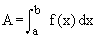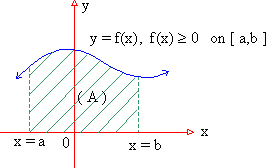Your browser does not support the IFRAME tag.Home MonkeyNotes Printable Notes Digital Library Study Guides Study Smart Parents Tips College Planning Test Prep Fun Zone Help / FAQ How to Cite New Title Request

 8.2    Area The area of the region bounded by a function y = f (x), the x-axis, and the two vertical boundaries i.e. ordinates x = a and x = b, can be determined by evaluating the definite integral(1) If the f (x) ³ 0 on [ a,b ], then the areai.e. area of the region below the graph y = f (x), above x-axis and between the ordinates x = a and x = b isIndex Chapter 1